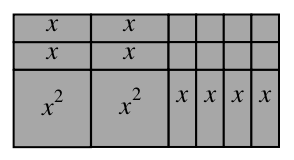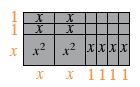### Home > CCA > Chapter 3 > Lesson 3.2.2 > Problem3-49

3-49.

Write the area of the rectangle at right as a product and as a sum.Find the height and the base by finding the dimensions of each row and column.$\text{height}=x+2\ \ \ \ \ \ \ \ \ \ \text{base}=2x+4$

To find the area as a product, $\text{area} = \text{base} × \text{height}$.

$\text{area} = (2x + 4)(x + 2)$

To express the area as a sum, add up all of the tiles and simplify.

$x + x + 1 + 1 + 1 + 1 + x + x + 1 + 1 + 1 + 1 + \\x² + x² + x + x + x + x = 2x² + 8x + 8$

$\text{Area as a product}=(2x+4)(x+2)$
$\text{Area as a sum} = 2x² + 8x + 8$